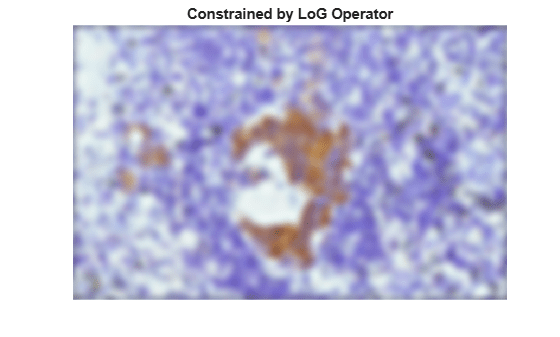# Deblur Images Using Regularized Filter

This example shows how to use regularized deconvolution to deblur images. Regularized deconvolution can be used effectively when limited information is known about the additive noise and constraints (such as smoothness) are applied on the recovered image. The blurred and noisy image is restored by a constrained least square restoration algorithm that uses a regularized filter.

### Simulate Gaussian Blur and Gaussian Noise

Read and display a pristine image that does not have blur or noise.

```I = im2double(imread('tissue.png')); imshow(I); title('Original Image'); text(size(I,2),size(I,1)+15, ... 'Image courtesy of Alan Partin, Johns Hopkins University', ... 'FontSize',7,'HorizontalAlignment','right');```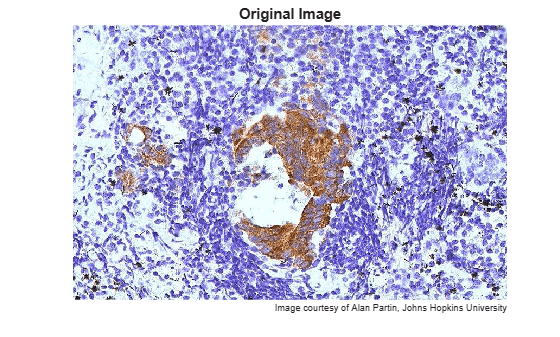Simulate a blurred image that might result from an out-of-focus lens. First, create a point-spread function, `PSF`, by using the `fspecial` function and specifying a Gaussian filter of size 11-by-11 and standard deviation 5. Then, convolve the point-spread function with the image by using `imfilter`.

```PSF = fspecial('gaussian',11,5); blurred = imfilter(I,PSF,'conv');```

Add zero-mean Gaussian noise to the blurred image by using the `imnoise` function.

```noise_mean = 0; noise_var = 0.02; blurred_noisy = imnoise(blurred,'gaussian',noise_mean,noise_var);```

Display the blurred noisy image.

```imshow(blurred_noisy) title('Blurred and Noisy Image')```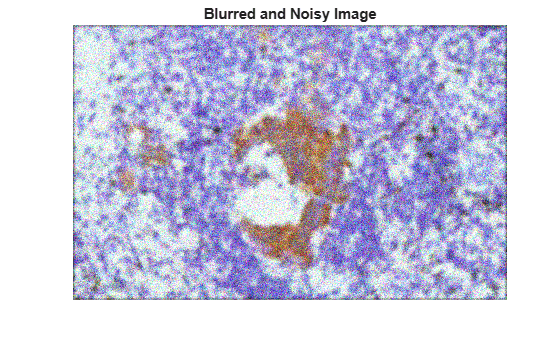### Restore Image Using Estimated Noise Power

Restore the blurred image by using the `deconvreg` function, supplying the noise power (NP) as the third input parameter. To illustrate how sensitive the algorithm is to the value of noise power, this example performs three restorations.

For the first restoration, use the true NP. Note that the example outputs two parameters here. The first return value, `reg1`, is the restored image. The second return value, `lagra`, is a scalar Lagrange multiplier on which the regularized deconvolution has converged. This value is used later in the example.

```NP = noise_var*numel(I); [reg1,lagra] = deconvreg(blurred_noisy,PSF,NP); imshow(reg1) title('Restored with True NP')```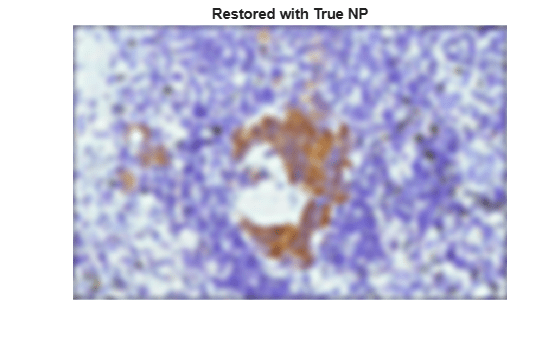For the second restoration, use a slightly overestimated noise power. The restoration has poor resolution.

```reg2 = deconvreg(blurred_noisy,PSF,NP*1.3); imshow(reg2) title('Restored with Larger NP')```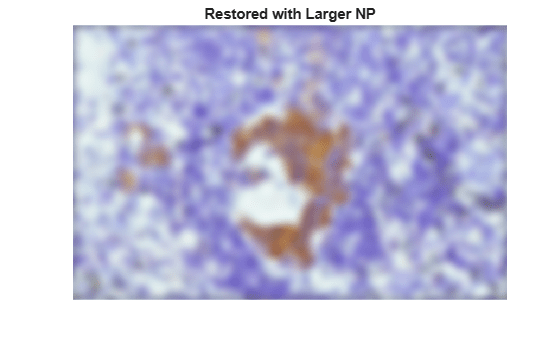For the third restoration, use a slightly underestimated noise power. The restoration has overwhelming noise amplification and ringing from the image borders.

```reg3 = deconvreg(blurred_noisy,PSF,NP/1.3); imshow(reg3) title('Restored with Smaller NP')```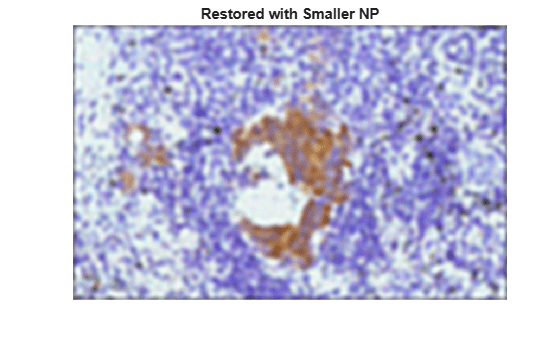### Reduce Noise Amplification and Ringing

You can reduce the noise amplification and ringing along the boundary of the image by calling the `edgetaper` function prior to deconvolution. The image restoration becomes less sensitive to the noise power parameter.

```Edged = edgetaper(blurred_noisy,PSF); reg4 = deconvreg(Edged,PSF,NP/1.3); imshow(reg4) title('Restored with Smaller NP and Edge Tapering')```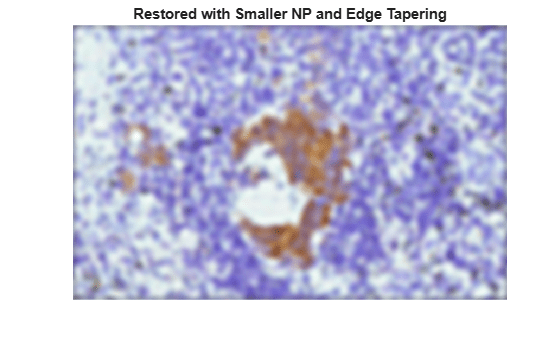### Use Lagrange Multiplier

Restore the blurred and noisy image, assuming that the optimal solution is already found and the corresponding Lagrange multiplier is known. In this case, any value passed for noise power, NP, is ignored.

To illustrate how sensitive the algorithm is to the Lagrange multiplier, this example performs three restorations. The first restoration uses the `lagra` output from the `reg1` restoration performed earlier.

```reg5 = deconvreg(Edged,PSF,[],lagra); imshow(reg5) title('Restored with LAGRA')```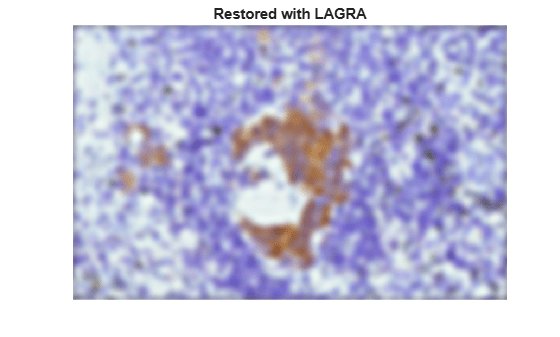The second restoration uses 100*`lagra` which increases the significance of the constraint. By default, this leads to oversmoothing of the image.

```reg6 = deconvreg(Edged,PSF,[],lagra*100); imshow(reg6) title('Restored with Large LAGRA')```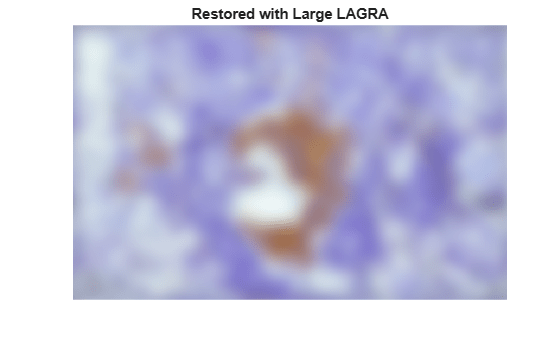The third restoration uses `lagra`/100 which weakens the constraint (the smoothness requirement set for the image). It amplifies the noise. For the extreme case when the Lagrange multiplier equals 0, the reconstruction is a pure inverse filtering.

```reg7 = deconvreg(Edged,PSF,[],lagra/100); imshow(reg7) title('Restored with Small LAGRA')```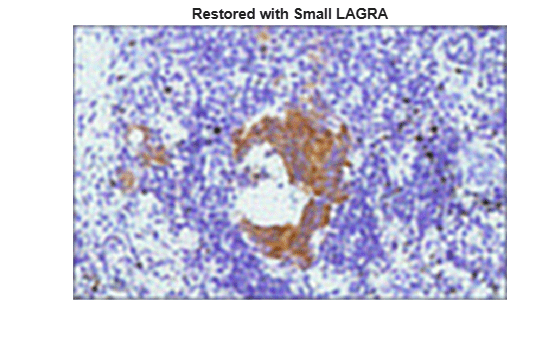### Use Different Smoothness Constraint

Restore the blurred and noisy image using a different constraint for the regularization operator. Instead of using the default Laplacian constraint on image smoothness, constrain the image smoothness only in one dimension (1-D Laplacian).

```regop = [-1 2 -1]; reg8 = deconvreg(blurred_noisy,PSF,[],lagra,regop); imshow(reg8) title('Constrained by 1-D Laplacian')```